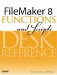# Truncate()

Category: Number

 Syntax: truncate ( number; precision )

Parameters:

numberAny expression that resolves to a numeric value.

precisionAny expression that resolves to a numeric value.

Data type returned: Number

Description:

Returns the specified number truncated to the specified number of decimal places (precision). Unlike the Round() function, the TRuncate() function simply discards further digits without performing any sort of rounding.

Truncating a number by using a precision parameter of 0 has the same effect as taking the Int() of that number. truncate (x; 0) = Int (x).

Negative values can be used for the precision parameter in the truncate() function. For instance, TRuncate (1234.1234; -1) returns 1230. truncate (1234.1234; -2) returns 1200.

Examples:

Function

Results

truncate (Pi; 6)

Returns 3.141592.

truncate (Amount; 2)

Returns 54.65 when Amount contains 54.651259.

truncate (1234.1234; 0)

Returns 1234.

truncate (-1234.1234; 0)

Returns 1234.FileMaker 8 Functions and Scripts Desk Reference
ISBN: 0789735113
EAN: 2147483647
Year: 2004
Pages: 352